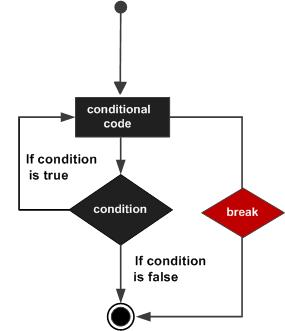# Lua - break Statement

When the break statement is encountered inside a loop, the loop is immediately terminated and the program control resumes at the next statement following the loop.

If you are using nested loops (i.e., one loop inside another loop), the break statement will stop execution of the innermost loop and start executing the next line of code after the block.

## Syntax

The syntax for a break statement in Lua is as follows −

```break
```

## Flow Diagram## Example

```--[ local variable definition --]
a = 10

--[ while loop execution --]
while( a < 20 )
do
print("value of a:", a)
a=a+1

if( a > 15)
then
--[ terminate the loop using break statement --]
break
end

end
```

When you build and run the above code, it produces the following result.

```value of a:	10
value of a:	11
value of a:	12
value of a:	13
value of a:	14
value of a:	15
```
lua_loops.htm